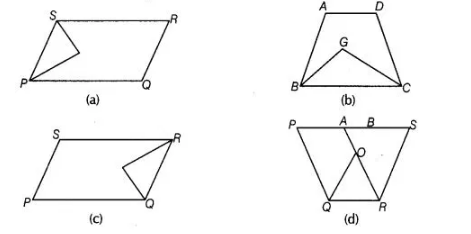# In which of the following figures,`
Question:

In which of the following figures, you find two polygons on the same base and between the same parallels?Solution:

(d) In figures (a), (b) and (c) there are two polygons on the same base but they are not between the same parallels.

In figure (d), there are two polygons (PQRA and BQRS) on the same base and between the same parallels .